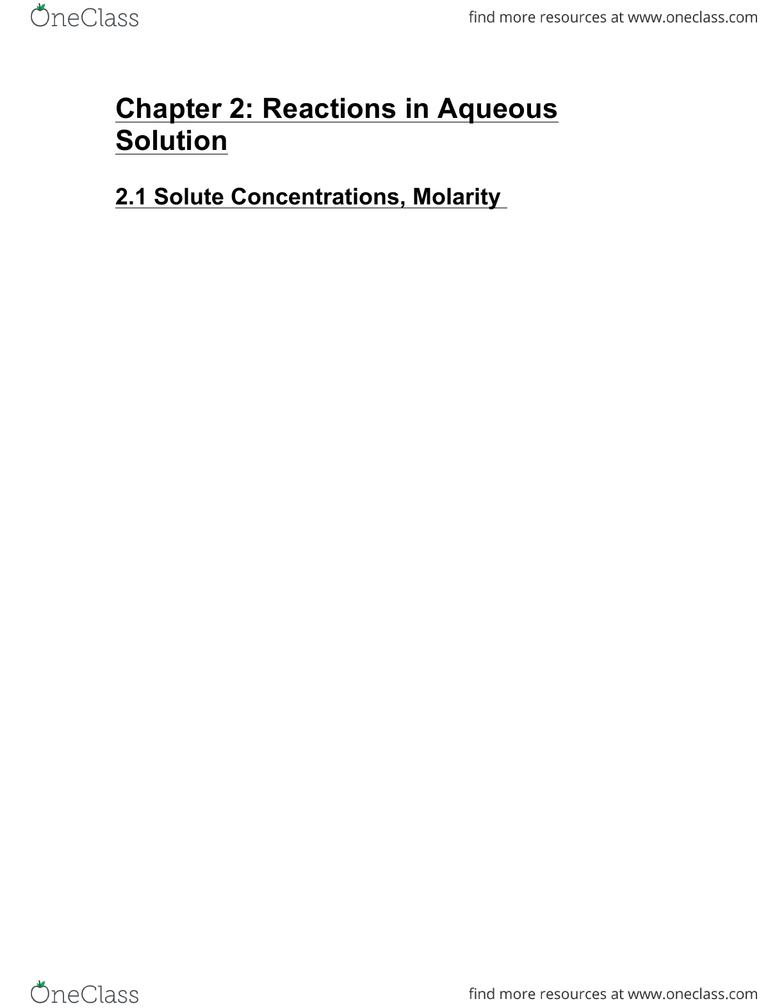Textbook Notes (270,000)
CA (160,000)
Western (10,000)
CHEM (200)
Chapter 2

Chemistry 1024A/B Chapter 2: Chem1024b_2013_chapter02.pdf

Department
Chemistry
Course Code
CHEM 1024A/B
Professor
Robert Klassen
Chapter
2

Page:
of 3144
Chapter 2: Reactions in Aqueous
Solution
2.1 Solute Concentrations, Molarity MHw, 3.1
Most chemical reactions that you will encounter occur
in solution (e.g. in your blood or cytoplasm, or in chemical reactors).
The number of moles of solute per volume of solution
is expressed in terms of its concentration, another
term for this is molarity.
concentration = moles of solute / liters of solution
C = n / V
MHw uses M "molarity" instead of C "concentration"
The concentration (=molarity) has units of moles per
liter (unit M).
1 M = 1 mol L-1
A 6 M solution of NaOH has 6 moles of NaOH per liter
of liquid.
[ ]s are often used in this context.
[NaOH] means “the concentration of NaOH”
In this example, [NaOH] = 6 M. We say “the solution
is 6 molar”.
Solutions can be concentrated (high conc.), e.g. 6 M
NaOH, or dilute (low conc.), e.g. 10-3M NaOH = 3 mM
NaOH.
45
Here is how you prepare a 0.1 M solution:
MHw, Fig 3.3
46
Calculating with concentrations
You have a bottle labeled “concentrated HCl”. It has
[HCl] = 12.0 M.
How many moles are there in 25.0 mL?
What volume of the 12 M solution must be taken to
contain 1.00 mol HCl?
Example: Oct. 2008 exam